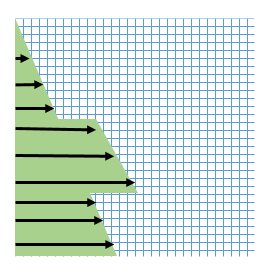# Python BC for M simulations: heterogeneous stress?

Hi all,

I am following the provided examples to define heterogeneous Neumann BC. I would like to define such a boundary condition for the horizontal stress component, based on the help of a python script:I have two questions.

1. The python script is accepted by OGS as follows:

class BCRight_sigma(OpenGeoSys.BoundaryCondition):
def getFlux(self, t, coords, primary_vars):
x, y, z = coords
Jac = [0.0,0.0,0.0] # value does not depend on primary variable
return (True, value, Jac)

where grad_sigma is a function that returns

` return something*(-y), something_different*y`

Has anyone experience with this approach? I already obtained corresponding IC thanks to constrained displacement BCs, now I would like to match these IC with meaningful BC, replacing the constrained displacement with constrained stresses.
To provide the full stress tensor as BC, is it enough to give the different components in the .prj file?

1. How do I access material ID from the python scripts, is there a quick way, similar to coords for the spatial coordinates?

Hi,

for the second question, the material ids are not available on the python side. I’m also unsure how to implement this quickly. But the integration point coordinates are available. If the mapping x → materialID is simple you could directly use it (for particular problem).

For the first question I have no answer for now, just discussion. You can (have to) specify on which component of displacement the BC is applied, so you would have 3 BCs for displacement (in 3d). But the shape function used for the boundary element will be for 2d, so you would return a 2-vector from python…

– d

Hi Luca. Regarding the first question: the full stress tensor is no boundary condition, rather the traction vector (so the normal stress and the two shear stresses on the surface in 3D (one in 2D)). If you calculate that boundary traction vector, it has components in x, y (and z). These, you can specify as Neumann bc for the components analogous to displacement.